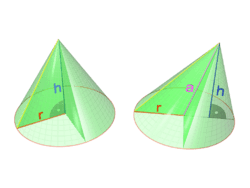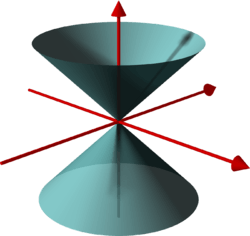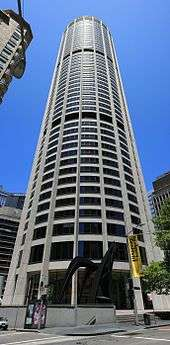# Cone

Not to be confused with Conical surface. For other uses, see Cone (disambiguation).A right circular cone and an oblique circular coneA double cone (not shown infinitely extended)

A cone is a three-dimensional geometric shape that tapers smoothly from a flat base (frequently, though not necessarily, circular) to a point called the apex or vertex.

A cone is formed by a set of line segments, half-lines, or lines connecting a common point, the apex, to all of the points on a base that is in a plane that does not contain the apex. Depending on the author, the base may be restricted to be a circle, any one-dimensional quadratic form in the plane, any closed one-dimensional figure, or any of the above plus all the enclosed points. If the enclosed points are included in the base, the cone is a solid object; otherwise it is a two-dimensional object in three-dimensional space. In the case of a solid object, the boundary formed by these lines or partial lines is called the lateral surface; if the lateral surface is unbounded, it is a conical surface.

In the case of line segments, the cone does not extend beyond the base, while in the case of half-lines, it extends infinitely far. In the case of lines, the cone extends infinitely far in both directions from the apex, in which case it is sometimes called a double cone. Either half of a double cone on one side of the apex is called a nappe.

The axis of a cone is the straight line (if any), passing through the apex, about which the base (and the whole cone) has a circular symmetry.

In common usage in elementary geometry, cones are assumed to be right circular, where circular means that the base is a circle and right means that the axis passes through the centre of the base at right angles to its plane. If the base is right circular the intersection of a plane with this surface is a conic section. In general, however, the base may be any shape and the apex may lie anywhere (though it is usually assumed that the base is bounded and therefore has finite area, and that the apex lies outside the plane of the base). Contrasted with right cones are oblique cones, in which the axis passes through the centre of the base non-perpendicularly.

A cone with a polygonal base is called a pyramid.

Depending on the context, "cone" may also mean specifically a convex cone or a projective cone.

Cones can also be generalized to higher dimensions.

## Further terminology

The perimeter of the base of a cone is called the "directrix", and each of the line segments between the directrix and apex is a "generatrix" or "generating line" of the lateral surface. (For the connection between this sense of the term "directrix" and the directrix of a conic section, see Dandelin spheres.)

The "base radius" of a circular cone is the radius of its base; often this is simply called the radius of the cone. The aperture of a right circular cone is the maximum angle between two generatrix lines; if the generatrix makes an angle θ to the axis, the aperture is 2θ.

A cone with a region including its apex cut off by a plane is called a "truncated cone"; if the truncation plane is parallel to the cone's base, it is called a frustum. An "elliptical cone" is a cone with an elliptical base. A "generalized cone" is the surface created by the set of lines passing through a vertex and every point on a boundary (also see visual hull).

## Measurements and equations

### Volume

The volumeof any conic solid is one third of the product of the area of the baseand the heightIn modern mathematics, this formula can easily be computed using calculus – it is, up to scaling, the integralWithout using calculus, the formula can be proven by comparing the cone to a pyramid and applying Cavalieri's principle – specifically, comparing the cone to a (vertically scaled) right square pyramid, which forms one third of a cube. This formula cannot be proven without using such infinitesimal arguments – unlike the 2-dimensional formulae for polyhedral area, though similar to the area of the circle – and hence admitted less rigorous proofs before the advent of calculus, with the ancient Greeks using the method of exhaustion. This is essentially the content of Hilbert's third problem – more precisely, not all polyhedral pyramids are scissors congruent (can be cut apart into finite pieces and rearranged into the other), and thus volume cannot be computed purely by using a decomposition argument.

### Center of mass

The center of mass of a conic solid of uniform density lies one-quarter of the way from the center of the base to the vertex, on the straight line joining the two.

### Right circular cone

#### Volume

For a circular cone with radius R and height H, the formula for volume becomeswhere r is the radius of the cone at height h measured from the apex:Thusand so#### Surface area

The lateral surface area of a right circular cone iswhereis the radius of the circle at the bottom of the cone andis the lateral height (the length of a line segment from the apex of the cone along its side to its base) of the cone (given by the Pythagorean theoremwhereis the height of the cone). The surface area of the bottom circle of a cone is the same as for any circle,. Thus, the total surface area of a right circular cone can be expressed as each of the following:(the area of the base plus the area of the lateral surface; the termis the slant height)whereis the radius andis the height.whereis the radius andis the lateral height.
• Circumference and lateral heightwhereis the circumference andis the lateral height.
• Apex angle and heightwhereis the apex angle andis the height.

#### Equation form

A right solid circular cone with heightand aperture, whose axis is thecoordinate axis and whose apex is the origin, is described parametrically aswhererange over,, and, respectively.

In implicit form, the same solid is defined by the inequalitieswhereMore generally, a right circular cone with vertex at the origin, axis parallel to the vector, and aperture, is given by the implicit vector equationwhereorwhere, anddenotes the dot product.

### Elliptic cone

In the Cartesian coordinate system, an elliptic cone is the locus of an equation of the form## Projective geometryIn projective geometry, a cylinder is simply a cone whose apex is at infinity, which corresponds visually to a cylinder in perspective appearing to be a cone towards the sky.

In projective geometry, a cylinder is simply a cone whose apex is at infinity. Intuitively, if one keeps the base fixed and takes the limit as the apex goes to infinity, one obtains a cylinder, the angle of the side increasing as arctan, in the limit forming a right angle. This is useful in the definition of degenerate conics, which require considering the cylindrical conics.

## Higher dimensions

The definition of a cone may be extended to higher dimensions (see convex cones). In this case, one says that a convex set C in the real vector space Rn is a cone (with apex at the origin) if for every vector x in C and every nonnegative real number a, the vector ax is in C. In this context, the analogues of circular cones are not usually special; in fact one is often interested in polyhedral cones.Wikimedia Commons has media related to Cone (geometry).